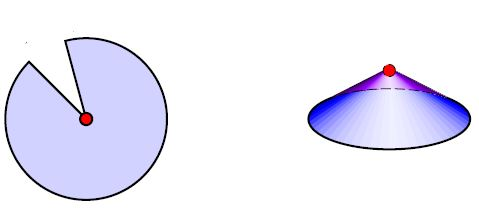nLab cone (Riemannian geometry)

Contents

For other, related, concepts of a similar name see at cone.

Context

Riemannian geometry

Riemannian geometry

Contents

Idea

In (pseudo-)Riemannian geometry, a cone is a part of a (pseudo-)Riemannian manifold where the metric tensor is locally of the form $d s^2 = d r^2 + r^2 d s^2_1$. The point that would correspond to $r = 0$ is the “conical singularity”.

Examples

Spherical cones

The metric cone on the round sphere is simply Euclidean space

$C(S^n) \simeq \mathbb{R}^{n+1} \setminus \{0\}$

and hence may in fact be continued non-singularly also at the cone tip.

For $G$ a finite group with a free action on the round sphere $S^n$, the quotient space $S^n/G$ exists as a Riemannian manifold. The metric cone $C(S^n/G)$ on this is singular at the origin as soon as $G$ is not the trivial group.

If here $G = \mathbb{Z}_n$ is a cyclic group one says that this cone is obtained from flat Euclidean space by introducing a “deficit angle”.If one passes beyond smooth manifolds to orbifolds, then the cone tip in $C(S^n/G)$ may be included. The result is the orbifold $\mathbb{R}^{n+1}\sslash G$ which is the homotopy quotient of Euclidean space by the linear $G$-action ($G$-representation).

Such conical singularities appear for instance in the far-horizon geometry of BPS black branes. Special cases are ADE-singularities.

$G_2$-manifolds

Three examples of cones admitted by simply-connected G2-manifolds seem to be known: cones on $\mathbb{C}P^3$, $SU(3)/U(1)\times U(1)$ and $S^3 \times S^3$. (Atiyah-Witten 01)

Discussion in the context of 3-manifolds and orbifolds:

• Daryl Cooper, Craig Hodgson, Steve Kerckhoff, Three-dimensional Orbifolds and Cone-Manifolds, MSJ Memoirs Volume 5, 2000 (pdf, euclid:1389985812)

Discussion of supergravity black brane-solutions at conical singularities (cone branes) includes the following (see also at far-horizon geometry)